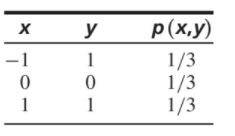×
Get Full Access to Statistics For Engineers And Scientists - 4 Edition - Chapter 2.6 - Problem 22e
Get Full Access to Statistics For Engineers And Scientists - 4 Edition - Chapter 2.6 - Problem 22e

×

# Here are two random variables that are uncorrelated butISBN: 9780073401331 38

## Solution for problem 22E Chapter 2.6

Statistics for Engineers and Scientists | 4th Edition

• Textbook Solutions
• 2901 Step-by-step solutions solved by professors and subject experts
• Get 24/7 help from StudySoup virtual teaching assistantsStatistics for Engineers and Scientists | 4th Edition

4 5 1 401 Reviews
24
1
Problem 22E

Here are two random variables that are uncorrelated but not independent. Let X and Y have the following joint probability mass function:a. Use the definition of independence on page 141 to show that X and Y are not independent (in fact $$Y=|X|$$, so Y is actually a function of X).

b. Show that X and Y are uncorrelated.

Equation Transcription:Text Transcription:

p(x,y)

Y=|X|

Step-by-Step Solution:

Step 1 of 3:

Given, let X and Y have the joint probability mass function.

 x y p(x,y) -1 1 1/3 0 0 1/3 1 1 1/3

Step 2 of 3

Step 3 of 3

##### ISBN: 9780073401331

Statistics for Engineers and Scientists was written by and is associated to the ISBN: 9780073401331. The answer to “?Here are two random variables that are uncorrelated but not independent. Let X and Y have the following joint probability mass function: a. Use the definition of independence on page 141 to show that X and Y are not independent (in fact $$Y=|X|$$, so Y is actually a function of X).b. Show that X and Y are uncorrelated.Equation Transcription:Text Transcription:p(x,y)Y=|X|” is broken down into a number of easy to follow steps, and 60 words. The full step-by-step solution to problem: 22E from chapter: 2.6 was answered by , our top Statistics solution expert on 06/28/17, 11:15AM. Since the solution to 22E from 2.6 chapter was answered, more than 399 students have viewed the full step-by-step answer. This full solution covers the following key subjects: function, uncorrelated, show, independent, let. This expansive textbook survival guide covers 153 chapters, and 2440 solutions. This textbook survival guide was created for the textbook: Statistics for Engineers and Scientists , edition: 4.

## Discover and learn what students are asking

Calculus: Early Transcendental Functions : Product and Quotient Rules and Higher-Order Derivatives
?Using the Product Rule In Exercises 1–6, use the Product Rule to find the derivative of the function. $$h(t)=\sqrt{t}\left(1-t^{2}\right)$$

Statistics: Informed Decisions Using Data : The Design of Experiments
?Explain the difference between a single-blind and a double- blind experiment.

Unlock Textbook Solution